# 7.4 Additional Properties of Hyperbolas

Let C be the hyperbola with equation x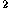/a-y/b=1, and let F,F'=(±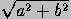,0) be its foci (see Figure 7.1.3 ). The conjugate hyperbola of C is the hyperbola C' with equation -x/a+y/b=1. It has the same asymptotes as C, the same axes (transverse and conjugate axes being interchanged), and its eccentricity e' is related to that of C by 1/e'+1/e=1.

• A parametric representation for C is (a sec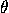, b tan).

A different parametric representation, which gives one branch only, is

(a cosh, b sinh):The area of the shaded sector above is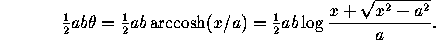The length of the arc from (a,0) to the point

(a cosh, b sinh)

is given by the elliptic integral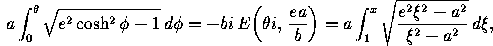where e is the eccentricity,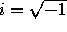, and x=a cosh. (See the Standard Math Tables and Formulas for elliptic integrals.)

• A rational parametric representation for C is given by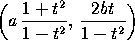.
• The polar equation for C in the usual polar coordinate system is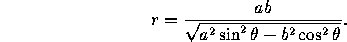With respect to a system with origin at a focus the equation is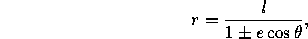where l=b/a is half the latus rectum. (Use the - sign for the focus with positive x-coordinate and the + sign for the other.)

• Let P be any point of C. The unsigned difference between the distances PF and PF' is constant, and equal to 2a.
• Let P be any point of C. Then the rays PF and PF' make the same angle with the tangent to C at P. Thus any light ray originating at F and reflected in the hyperbola will appear to emanate from F'.
• Let T be any line tangent to C. The product of the distances from F and F' to T is constant, and equals b.
• Let P be any point of C. The area of the parallelogram formed by the asymptotes and the parallels to the asymptotes going through P is constant, and equals ½ab.
• Let L be any line in the plane. If L intersects C at P and P', and intersects the asymptotes at Q and Q', the distances PQ and P'Q' are the same. If L is tangent to C we have P=P', so the point of tangency bisects the segment QQ'.

Next: 7.5 Additional Properties of Parabolas
Up: 7 Conics
Previous: 7.3 Additional Properties of EllipsesThe Geometry Center Home Page

Silvio Levy
Wed Oct 4 16:41:25 PDT 1995

This document is excerpted from the 30th Edition of the CRC Standard Mathematical Tables and Formulas (CRC Press). Unauthorized duplication is forbidden.